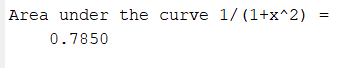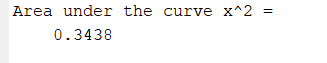Open in App
Not now

# MATLAB – Trapezoidal numerical integration without using trapz

• Difficulty Level : Medium
• Last Updated : 04 Jul, 2021

Trapezoidal rule is utilized to discover the approximation of a definite integral. The main idea in the Trapezoidal rule is to accept the region under the graph of the given function to be a trapezoid rather than a rectangle shape and calculate its region.

The formula for numerical integration using trapezoidal rule is:where h = (b-a)/n

Now we take an example for calculating the area under the curveusing 10 subintervals.

Example:

## Matlab

 `% MATLAB program for calculate the  ` `% area under the curve ∫_0^11/(1+x^2) dx  ` `% using 10 subintervals specify the variable ` `% x as symbolic ones The syms function creates  ` `% a variable dynamically and automatically assigns ` `% to a MATLAB variable with the same name ` `syms x ` ` `  `% Lower Limit ` `a=0;   ` ` `  `% Upper Limit ` `b=1;    ` ` `  `% Number of segments ` `n=10;  ` ` `  `% Declare the function ` `f1=1/(1+x^2); ` ` `  `% inline creates a function of ` `% string containing in f1 ` `f=inline(f1);  ` ` `  `% h is the segment size ` `h=(b - a)/n; ` ` `  `% X stores the summation of first ` `% and last segment ` `X=f(a)+f(b); ` ` `  `% variable R stores the summation of ` `% all the terms from 1 to n-1 ` `R=0; ` `for` `i = 1:1:n-1 ` `    ``xi=a+(i*h); ` `    ``R=R+f(xi); ` `end` ` `  `% Formula to calculate numerical integration ` `% using Trapezoidal Rule ` `I=(h/2)*(X+2*R); ` ` `  `% Display the output ` `disp(``'Area under the curve 1/(1+x^2) = '``); ` `disp(I);`

Output:Let’s take another example for calculating the area under the curveusing 4 subintervals.

Example:

## Matlab

 `% MATLAB program for calculate ` `% the area under the curve∫_0^1x^2 dx     ` `% using 4 subintervals. ` `% specify the variable x as symbolic ones ` ` `  `syms x ` ` `  `% Lower Limit ` `a=0;  ` ` `  `% Upper Limit ` `b=1;  ` ` `  `% Number of segments ` `n=4;   ` ` `  `% Declare the function ` `f1=x^2; ` ` `  `% inline creates a function of ` `% string containing in f1 ` `f=inline(f1);  ` ` `  `% h is the segment size ` `h=(b - a)/n; ` `X=f(a)+f(b); ` ` `  `% variable R stores the summation  ` `% of all the terms from 1 to n-1 ` `R=0; ` `for` `i = 1:1:n-1 ` `    ``xi=a+(i*h); ` `    ``R=R+f(xi); ` `end` ` `  `% Formula to calculate numerical ` `% integration using Trapezoidal Rule ` `I=(h/2)*(X+2*R); ` ` `  `% Display the output ` `disp(``'Area under the curve x^2 = '``); ` `disp(I); `

Output:My Personal Notes arrow_drop_up
Related Articles